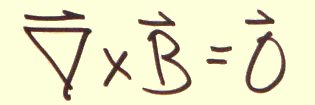# Electromagnetism

1. Derive the Euler-Lagrange equations, used to find field equations
2. EM invariants
3. Derive the Maxwell homogeneous equations
4. Derive the Maxwell source equations
5. Jupyter notebook derivation pdf ipynb